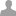## math functions ?

hello,

I'm very newby to C programming and I have some interrogation about the librairies

I need some mathematical function (log and exp mainly)

do I need a special librairy or ares these fonctions already in the main one ?

sorry for the basic question

Math functions are provided by math.h, which is included by default.

```  float x = exp(2.0);
float y = log(x);
printf("e^2 = %f, ln(e^2) = %f\n", x, y);
```

thank you a lot

is there a list of the librairies included by defaut ?  to avoid other silly questions ;-)

Hi Pion,

The standard c libraries we include by default within mbed.h are:

```#include <stdio.h>
#include <stdlib.h>
#include <string.h>
#include <math.h>
#include <time.h>
```

FYI, at some point I'd like us to specify the definitions of all these standard c functions on mbed, but for now the following is a good reference:

Hope that helps.

Simon

that definitly help

thank you a lot

Hi there!

I'm guessing with previous posts is that <ctype> library is not included?

I need to use the function isdigit() which is in that library...any suggestions?

thanks!

Use ctype.h instead.

or

#include <cctype>

with std::isdigit()

Hi, Thanks for the pointer to http://www.cplusplus.com/reference/clibrary/

I can't find M_PI in there, and the mbed compiler doesn't recognise PI or M_PI. I could just #define it locally, but I feel like I'm missing the 'clean' way to make nice code. Suggestions please?

Thanks in advance:

Has anyone checked the accuracy of the math functions?

I am getting log(100.0) equal to 4.605170. It should be 2.0000 log(10.0) = 2.302585, s/b 1.0000 log(1.0) = 0.000, s/b 0.000 yeaaa!!!!, this one is correct log(0.1) = -2.302585, s/b -1.0000 log(0.01) = -4.605170, s/b -2.0000

I've tried float(log(100.0)) and double(log(100.0)), but the answer is the same.

Am I doing something wrong or is log broken? ...kevin

Kevin -

The natural logarithm (ln) of 100 is 4.605170.

Perhaps the function you seek is log10().

Regards,

- Gary

log() returns the natural logarithm (ln), not base 10 (use log10 for that).

that was it, thanks

Are there many functions and defines missing in the mbed math library or am doing it wrong. Don't seem find lround, trunc, M_PI, etc...

Help appreciated.

####stefan he wrote:

Are there many functions and defines missing in the mbed math library or am doing it wrong. Don't seem find lround, trunc, M_PI, etc...

Help appreciated.

I seem to be hitting same problem...

But there is a solution. Put this in the header file of your project:

```#ifndef M_PI
#define M_PI           3.14159265358979323846
#endif

#ifndef lround
#define lround(var)    (long)(var+0.5f)
#endif
```

hello what function in MBED replaces the MAP function?

hi what function in MBED replaces the MAP function?

You mean the map function from Arduino? Just see: http://www.arduino.cc/en/Reference/Map. At the bottom there is the actual formula used by that function. It is a pretty simple one, so you can just copy that to your program and use it.